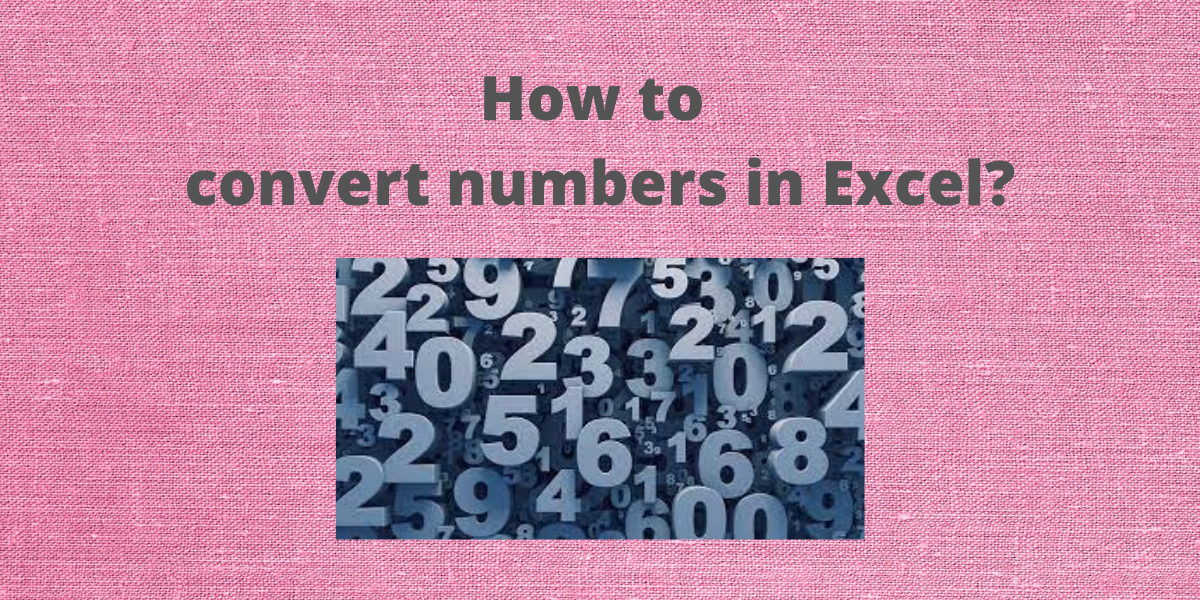# How to Convert Numbers in Excel – Step-By-StepOne may often require to convert numbers in Excel from one format to another e.g. decimal number 20 to Roman numerals XX etc. Also, from decimal to binary, octal and hexadecimal. Excel provides some inbuilt functions to help users convert numbers from one numbering system to another with ease.

## Convert Decimal to Roman Numbers in Excel

Roman numerals are based on the ancient Roman system where symbols are employed as numerical notations. I, V, X, L, C, D, and M, stand for 1, 5, 10, 50, 100, 500, and 1,000 respectively. Excel provides an inbuilt function ROMAN to convert decimal numbers to binary.

Syntax: ROMAN(n),

where n is the number or the cell reference of the cell containing the number. Follow these steps to convert decimal numbers to Roman numerals:

• Select the cell where you want to display the Roman numerals.
• Type =ROMAN(, select the cell containing the decimal number and finish the formula with ).
• Press the Enter key to display the result.

## Convert Decimal to Binary in Excel

Binary notation uses just two digits: 1 and 0 to represent numbers, where each bit represents power of 2. for example: number 9 is written as 1001 as 9 = 2^3 + 0 + 0 + 2^0 . Excel provides an inbuilt function DEC2BIN to convert decimal numbers to binary.

Syntax: DEC2BIN(n),

where n is the number you wish to convert or the cell reference of the cell containing the number. Follow these steps to convert decimal numbers to binary:

• Select the cell where you want to display the binary number.
• Type =DEC2BIN(, select the cell containing the decimal number and finish the formula with ).
• Press the Enter key to display the result.

Conversely, one can convert a given binary number to a decimal number using an inbuilt function BIN2DEC. Syntax: BIN2DEC(n), where n is the number you wish to convert or the cell reference of the cell containing the number. Follow these steps to convert binary to decimal numbers:

• Select the cell where you want to display the decimal number.
• Type =BIN2DEC(, select the cell containing the decimal number and finish the formula with ).
• Press the Enter key to display the result.

## Convert Decimal to Hexadecimal numbers in Excel

The hexadecimal number system is a 16-character numerical system (base 16). It uses the same decimal numbers from 0 to 9 and six extra symbols from the English alphabet A, B, C, D, E, and F for numbers 10, 11, 12, 13, 14, and 15 respectively. Excel provides an inbuilt function DEC2HEX to convert decimal numbers to hexadecimal.

Syntax: DEC2HEX(n),

where n is the number you wish to convert or the cell reference of the cell containing the number. Follow these steps to convert decimal numbers to hexadecimal:

• Select the cell where you want to display the hexadecimal number.
• Type =DEC2HEX(, select the cell containing the decimal number and finish the formula with ).
• Press the Enter key to display the result.

Conversely, one can convert a given hexadecimal number to a decimal number using an inbuilt function HEX2DEC. Syntax: HEX2DEC(n), where n is the number you wish to convert or the cell reference of the cell containing the number. Follow these steps to convert hexadecimal to decimal numbers:

• Select the cell where you want to display the decimal number.
• Type =HEX2DEC(, select the cell containing the decimal number and finish the formula with ).
• Press the Enter key to display the result.

## Convert Decimal to Octal numbers in Excel

The octal numeral system is a base-8 number system that uses the same decimal numbers from 0 to 7. Excel provides an inbuilt function DEC2OCT to convert decimal numbers to octal. Syntax: DEC2OCT(n), where n is the number you wish to convert or the cell reference of the cell containing the number. Follow these steps to convert decimal numbers to octal:

• Select the cell where you want to display the octal.
• Type =DEC2OCT(, select the cell containing the decimal number and finish the formula with ).
• Press the Enter key to display the result.

Conversely, one can convert given octal to a decimal number using an inbuilt function OCT2DEC. Syntax: OCT2DEC(n), where n is the number you wish to convert or the cell reference of the cell containing the number. Follow these steps to convert octal to decimal numbers:

• Select the cell where you want to display the decimal number.
• Type =OCT2DEC(, select the cell containing the decimal number and finish the formula with ).
• Press the Enter key to display the result.

## Conclusion

In this tutorial, we learned how to quickly and easily convert decimal numbers to their equivalent in other number systems such as roman numerals, binary, octal, and hexadecimal using inbuilt functions in Excel.